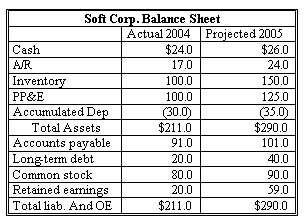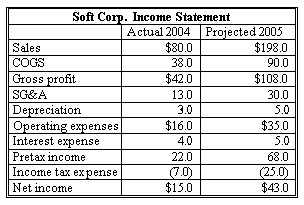### CFA Practice Question

There are 201 practice questions for this study session.

### CFA Practice QuestionSoft Corp.' FCFF should be ______.
A. -\$24.85.
B. -\$20.85.
C. -\$22.85.
Explanation: FCInv = \$125 - \$100 = \$25.
WC2005 = (\$24 + \$150) - (\$101) = \$73.
WC2004 = (\$17 + \$100) - (\$91) = \$26.
WCInv = \$73 - \$26 = \$47.
t = \$25 / \$68 = 37%.
FCFF = NI + NCC + [Int(1 - t)] - FCInv - WCInv = \$43 + \$5 + [\$5(1 - 0.37)] - \$25 - \$47 = -\$20.85.